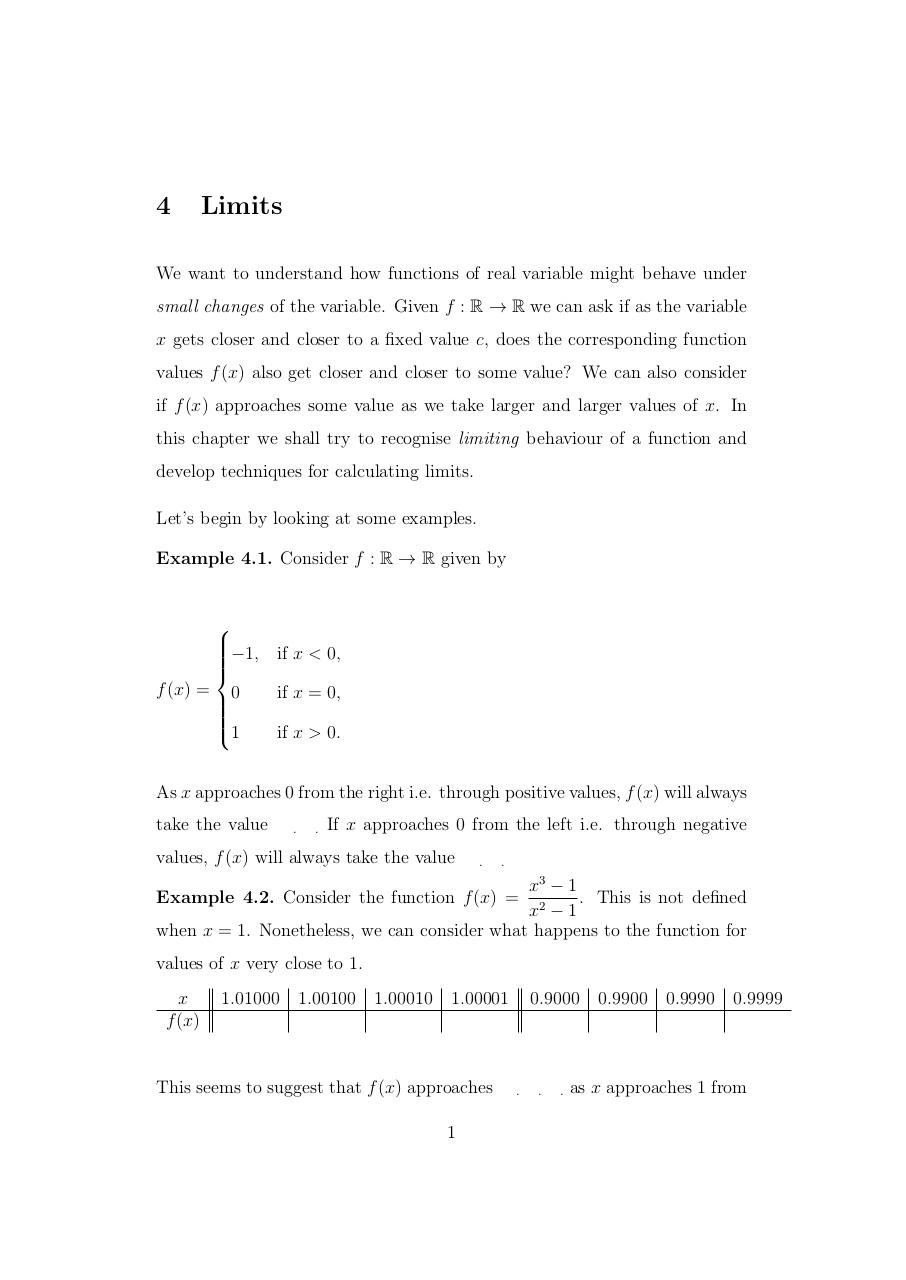# Limits.pdfPage 1 2 3 4 5 6 7 8 9 10 11 12

#### Text preview

4

Limits

We want to understand how functions of real variable might behave under
small changes of the variable. Given f : R → R we can ask if as the variable
x gets closer and closer to a fixed value c, does the corresponding function
values f (x) also get closer and closer to some value? We can also consider
if f (x) approaches some value as we take larger and larger values of x. In
this chapter we shall try to recognise limiting behaviour of a function and
develop techniques for calculating limits.
Let’s begin by looking at some examples.
Example 4.1. Consider f : R → R given by

−1, if x &lt; 0,

f (x) = 0
if x = 0,

1
if x &gt; 0.
As x approaches 0 from the right i.e. through positive values, f (x) will always
take the value

.

.

If x approaches 0 from the left i.e. through negative

values, f (x) will always take the value

.

.

x3 − 1
. This is not defined
x2 − 1
when x = 1. Nonetheless, we can consider what happens to the function for

Example 4.2. Consider the function f (x) =

values of x very close to 1.
x
f (x)

1.01000 1.00100 1.00010 1.00001

This seems to suggest that f (x) approaches
1

0.9000 0.9900 0.9990 0.9999

.

.

.

as x approaches 1 from